## Selina Concise Mathematics Class 10 ICSE Solutions Chapter 5 Quadratic Equations Ex 5A

These Solutions are part of Selina Concise Mathematics Class 10 ICSE Solutions. Here we have given Selina Concise Mathematics Class 10 ICSE Solutions Chapter 5 Quadratic Equations Ex 5A.

Other Exercises

Question 1.
Without solving, comment upon the nature of roots of each of the following equations:
(i) 7x² – 9x + 2 = 0
(ii) 6x² – 13x + 4 = 0
(iii) 25x² – 10x + 1 = 0
(iv) x² + 2√3 x – 9 = 0
(v) x² – ax – b² = 0
(vi) 2x² + 8x + 9 = 0
Solution: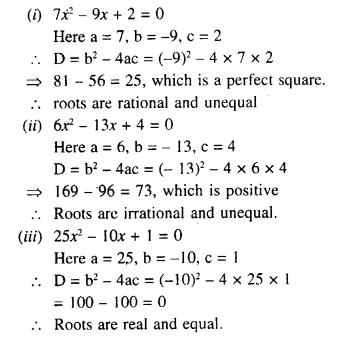Question 2.
Find the value of ‘p’, if the following quadratic equations have equal roots :
(i) 4x² – (p – 2) x + 1 = 0
(ii) x² + (p – 3) x + p = 0
Solution: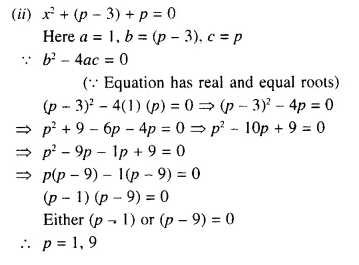Question 3.
The equation 3x² – 12x + (n – 5) = 0 has equal roots. Find the value of n.
Solution:
3x² – 12x + (n – 5) = 0
Here a = 3, b = -12, c = (n – 5)
D = b² – 4ac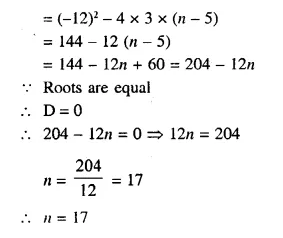Question 4.
Find the value of ‘m’, if the following equation has equal roots :
(m – 2) x² – (5 + m) x + 16 = 0
Solution: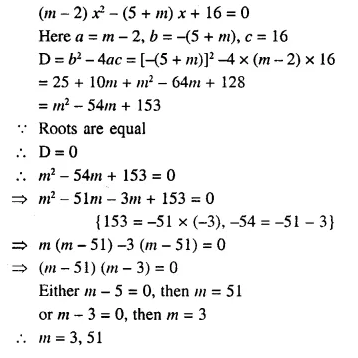Question 5.
Find the value of k for the which the equation 3x² – 6x + k = 0 has distinct and real root.
Solution: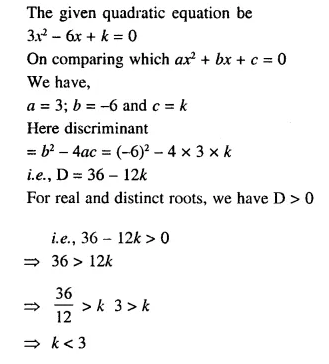Hope given Selina Concise Mathematics Class 10 ICSE Solutions Chapter 5 Quadratic Equations Ex 5A are helpful to complete your math homework.

If you have any doubts, please comment below. Learn Insta try to provide online math tutoring for you.